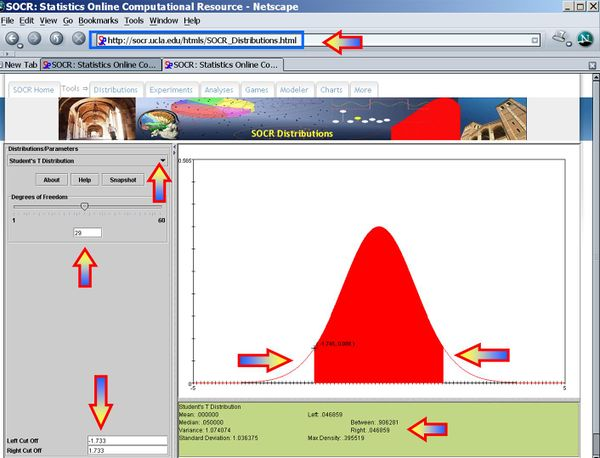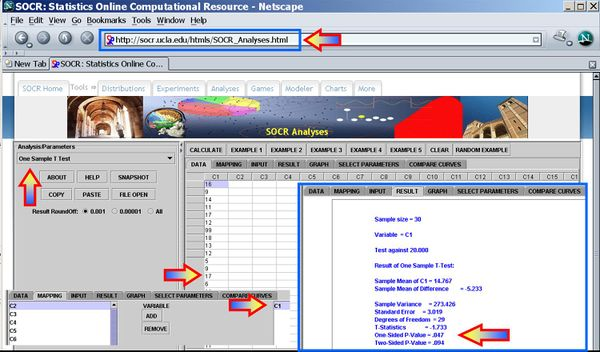# AP Statistics Curriculum 2007 Hypothesis L Mean

## General Advance-Placement (AP) Statistics Curriculum - Testing a Claim About a Mean: Large Samples

We already saw how to construct point and interval estimates for the population mean in the large sample case. Now, we show how to do hypothesis testing of the mean for large sample-sizes.

### Background

• Recall that for a random sample {$$X_1, X_2, X_3, \cdots , X_n$$} of the process, the population mean may be estimated by the sample average, $$\overline{X_n}={1\over n}\sum_{i=1}^n{X_i}$$.
• For a given small $$\alpha$$ (e.g., 0.1, 0.05, 0.025, 0.01, 0.001, etc.), the $$(1-\alpha)100%$$ Confidence interval for the mean is constructed by

$CI(\alpha): \overline{x} \pm z_{\alpha\over 2} E,$

where the margin of error E is defined as

$E = \begin{cases}{\sigma\over\sqrt{n}},& \texttt{for-known}-\sigma,\\ {{1\over \sqrt{n}} \sqrt{\sum_{i=1}^n{(x_i-\overline{x})^2\over n-1}}},& \texttt{for-unknown}-\sigma.\end{cases}$

and $$z_{\alpha\over 2}$$ is the critical value for a Standard Normal distribution at $${\alpha\over 2}$$.

### Hypothesis Testing About a Mean: Large Samples

• Null Hypothesis$H_o: \mu=\mu_o$ (e.g., $$\mu_o=0$$)
• Alternative Research Hypotheses:
• One sided (uni-directional)$H_1: \mu >\mu_o$, or $$H_1: \mu<\mu_o$$
• Double sided: $$H_1: \mu \not= \mu_o$$

#### Known Variance

$Z_o = {\overline{x} - \mu_o \over {\sigma \over \sqrt{n}}} \sim N(0,1)$.

#### Unknown Variance

$T_o = {\overline{x} - \mu_o \over SE(\overline{x})} = {\overline{x} - \mu_o \over {{1\over \sqrt{n}} \sqrt{\sum_{i=1}^n{(x_i-\overline{x})^2\over n-1}}})} \sim T_{(df=n-1)}$.

### Example

We want to test at $$\alpha=0.05$$
Null hypothesis$H_o: \mu=20$
Against a double-sided research alternative hypothesis$H_1: \mu \not= 20$.
 16 9 14 11 17 12 99 18 13 12 5 9 17 6 11 17 18 20 6 14 7 11 12 5 18 6 4 13 11 12

We had the following 2 sample statistics computed earlier $\overline{x}=\hat{\mu}=14.77$ $s=\hat{\sigma}=16.54$

As the population variance is not given, we have to use the T-Statistics$T_o = {\overline{x} - \mu_o \over SE(\overline{x})} \sim T(df=29)$ $T_o = {\overline{x} - \mu_o \over SE(\overline{x})} = {14.77 - 20 \over {{1\over \sqrt{30}} \sqrt{\sum_{i=1}^{30}{(x_i-14.77)^2\over 29}}})}=-1.733$. $P(T_{(df=29)} < T_o=-1.733)=0.047$, thus

the $$p-value=2\times 0.047= 0.094$$ for this (double-sided) test.

Therefore, we can not reject the null hypothesis at $$\alpha=0.05$$! The left and right white areas at the tails of the T(df=29) distribution depict graphically the probability of interest, which represents the strength of the evidence (in the data) against the Null hypothesis. In this case, the cumulative tail area is 0.094, which is larger than the initially set Type I error $$\alpha = 0.05$$ so we can not reject the null hypothesis.### Examples

#### Cavendish Mean Density of the Earth

A number of famous early experiments of measuring physical constants has later been shown to be biased. In the 1700's Henry Cavendish measured the Mean density of the Earth. Formulate and test null and research hypotheses about these data regarding the now known exact mean-density value = 5.517. These sample statistics may be helpful (you can also find the transposed table here):

n = 23, sample mean = 5.483, sample SD = 0.1904
 5.36 5.29 5.58 5.65 5.57 5.53 5.62 5.29 5.44 5.34 5.79 5.1 5.27 5.39 5.42 5.47 5.63 5.34 5.46 5.3 5.75 5.68 5.85

#### US Federal Budget Deficit

Use the US Federal Budget Deficit data (1849-2016) to formulate and test several Null hypotheses on whether the US Federal Budget Deficit is trivial ($$\mu_o=0$$) in different time frames (e.g., 1849-2000 or 1900-2016). Start with some exploratory data analyses to plot the data as shown in the data page. Then you can use the SOCR One Sample T-Test and the SOCR Confidence Interval applets. What are your conclusions?

### Hypothesis Testing Summary

Important parts of Hypothesis Test conclusions:

• Decision (significance or no significance)
• Parameter of Interest
• Variable of Interest
• Population under study
• (optional but preferred) P-value

### Parallels between Hypothesis Testing and Confidence Intervals

These are different methods for coping with the uncertainty about the true value of a parameter caused by the sampling variation in estimates.

• Confidence Intervals: A fixed level of confidence is chosen. We determine a range of possible values for the parameter that are consistent with the data (at the chosen confidence level).
• Hypothesis (Significance) testing: Only one possible value for the parameter, called the hypothesized value, is tested. We determine the strength of the evidence (confidence) provided by the data against the proposition that the hypothesized value is the true value.

"-----